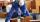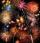# Pears

There were pears in the basket, I took two-fifths of them, and left six in the basket. How many pears did I take?

Result

x =  4

#### Solution:

h - x = 6
x = 2/5h

h-x = 6
2h-5x = 0

h = 10
x = 4

Calculated by our linear equations calculator.

Leave us a comment of example and its solution (i.e. if it is still somewhat unclear...):Be the first to comment!#### To solve this example are needed these knowledge from mathematics:

Need help calculate sum, simplify or multiply fractions? Try our fraction calculator. Do you have a linear equation or system of equations and looking for its solution? Or do you have quadratic equation?

## Next similar examples:

1. TreesFrom the total number of trees in the orchard, there are two-fifths pearls and apples are three eighty. The rest of the trees are 9 ceremonial. How many trees are in the set?
2. Boys and girlsThere are 28 girls in the hall. 5/7 of all children are boys. How many children and how many boys are there?
3. FruitsAmy bought a basket of fruits 1/5 of them were apples,1/4 were oranges, and the rest were 33 bananas. How many fruits did she buy in all?
4. ClassroomOne-eighth of 9th class was interested in studying at a grammar school, at a business academy one sixth, at secondary vocational schools quarter, to SOU one third and the remaining three students were interested in the school of art direction. How many st
5. Cleaning windowsCleaning company has to wash all the windows of the school. The first day washes one-sixth of the windows of the school, the next day three more windows than the first day and the remaining 18 windows washes on the third day. Calculate how many windows ha
6. Bricklayers8 bricklayers build a house for 630 days. How many bricklayers have to add after 150 days, so then the whole building completed in (next) 320 days?
7. King's birthdayTo celebrate the king's birthday workers fire 1/5 all purchased rockets. To celebrate the Queen's birthday fire 1/6 of the remaining rockets and to celebrate the birthday of king's son remaining 15,000 rockets. How many rockets they purchased?
8. Unknown numberI think the number - its sixth is 3 smaller than its third.
9. EqnSolve equation with fractions: 2x/3-50=40+x/4
10. Equation 15Solve equation with variables on both sides:
11. Unknown numberI think the number. I'll reduce it to its one-third. The result is then increased by one-third, and I get the number 12.
12. EquationSolve equation and check the result: 1.4x - 3/2 + x - 9,8 = x + 0,4/3 - 7 + 1,6/6
13. Find unknownFind unknown numerator: 4/8 + _/8 = 1
14. FractionsThree-quarters of an unknown number are 4/5. What is 5/6 of this unknown number?
15. Equation with fractionsSolve equation: ? It is equation with fractions.
16. Equation with xSolve the following equation: 2x- (8x + 1) - (x + 2) / 5 = 9
17. Simple equationSolve for x: 3(x + 2) = x - 18